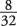## Relative Frequency Histogram

A relative frequency histogram uses the same information as a frequency histogram but compares each class interval to the total number of items. For example, the first interval (\$1 to \$5) contains 8 out of the total of 32 items, so the relative frequency of the first class interval is(see Table 1).The only difference between a frequency histogram and a relative frequency histogram is that the vertical axis uses relative or proportional frequency instead of simple frequency (see Figure 1).

Figure1 .Relative frequency histogram of items sold at a garage sale.